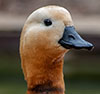# Getting started with the qacBase packageThe qacBase package contains functions and data sets designed to simplify data analyses and aid in the instruction of data science courses. The primary functions are described below.

## Preparing Data

Function Description
recodes recodes() provides a simple way to recode the values of numeric, character, or factor variables. See the vignette for examples.
standardize standardize() transforms all the numeric variables in a data frame to same mean and standard deviation (mean=0 sd=1, by default), without modifying character, factor, or dummy coded variables.
normalize normalize() transforms all the numeric variables in a data frame to same range of values ([0, 1] by default). Again, character and factor variables are left unchanged.

## Describing a data set

Function Description
contents contents() provides a comprehensive description of a data frame. The output is much more detailed than that provided by the base summary.data.frame() function, and is easier to read and understand. This function should be your first stop when looking at a new dataset.
df_plot dfPlot() helps you visual a data frame. Variable are grouped by type (numeric, integer, character, factor, date) and color coded. The percent of missing data for each variable is also displayed, along with the total number of variables and cases.
barcharts barcharts() provides bar charts of all the character or factor variables in a data frame, within a single graph.
histograms histograms() provides histograms of the all quantitative variables in a data frame, within a single graph.
densities densities() provides density charts of all the quantitative variables in a data frame, within a single graph.

## Exploratory data analysis

### Numeric variables

Function Description
qstats qstats() allows you to easily calculate any number of descriptive statistics (e.g., n, mean, sd) for a quantiatative variable. The results can be broken down by the levels of one of more categorical variables (groups). Any function that produces a single number can be used. See the vignette for examples.
univariate_plot univariatePlot() provides a detailed visualization of the distribution of values in a quantiative variable. The graph contains a histrogram, jittered dot plot, density curve, and boxplot, Annotations provide statistics such as n, mean, sd, median, min, max, skew, and outliers.
scatter scatter() generates a scatter plot and line of best fit with 95% confidence interval displaying the relationship between two quantiative variables. Annotations include the slope, correlation coefficient (r), r-squared, and p_value. Oultiers (determinded by studentized residuals) are flagged. Optionally, marginal distributions (histograms, boxplots, density curves, violin plots) can be added to the margins of the plot.
cor_plot corplot() plots the correlations among numeric variables in a data frame. Variables can be sorted to place variables with similar correlation patterns together.
groupdiff groupdiff() compares groups on a quantitative outcome using either a parametric (ANOVA) or nonparametric (Kruskal-Wallis) test. Summary statistics, pair-wise group differences (post-hoc comparisons), and plots are provided.

### Categorical Variables

Function Description
tab tab() generates a frequency table and bar chart for a categorical variable. There are many options including sorting categories by frequency, adding cumulative frequencies and percents, and combining infrequent categories into an ‘Other’ category. See the vignette for examples.
crosstab crosstab() generates a two-way frequency table from two categorical variables. There are many options including cell, row, and column percents, plotting options, and a chi-square test of independence. See the vignette for examples.